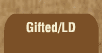Mathematics and Dyslexia

Tips for Learning the Times Tables

Tools for the Times Tables & Workbook

Home > Math > times tables practice > Sixes (big) in order

## The Six Times Tables In Order - second set

The digits of the numbers that you can divide by three always add up to a number three will go into. Even numbers always end in 2, 4, 6, 8, or 0. If both of those are true, then 6 will go into the number.

1. 6 x 6=
2. 6 x 7=
3. 6 x 8=
4. 6 x 9=
5. 6 x 10=
6. 10 x 6=
7. 9 x 6=
8. 8 x 6=
9. 7 x 6=
10. 6 x 6=
11. 7 x 6=
12. 6 x 8=
13. 9 x 6=
14. 6 x 6=
15. 8 x 6=